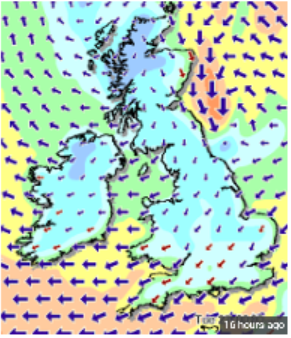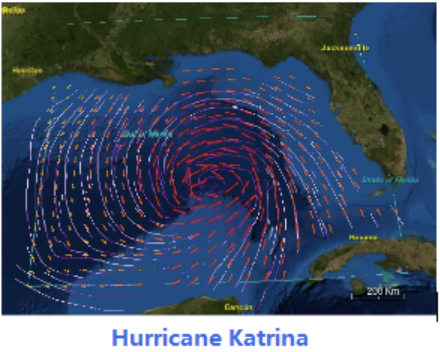# Trigonometry

## Chapter9Vectors

• $$\displaystyle \blert{\textbf{Geometric Form}}$$
• $$\displaystyle \blert{\textbf{Coordinate Form}}$$
• $$\displaystyle \blert{\textbf{The Dot Product}}$$A vector is a mathematical tool that indicates both a direction and a size, or magnitude. Vectors are often represented visually as arrows. For example, we can use vectors to indicate the speed and direction of the wind. You may see weather maps like the ones below.Usually the magnitude of the vector, (the wind speed in this example), is shown by the length of the arrow, but sometimes a color key makes the map easier to read.
Of particular interest are the winds produced by tropical storms, or hurricanes and the forces that create the storm. Near the Earth’s surface, winds spiral towards the center of a hurricane. They rotate in a counterclockwise direction in the Northern Hemisphere and in a clockwise direction in the Southern Hemisphere.
These rotating winds are called the hurricane’s primary circulation. A hurricane’s primary circulation involves four main forces: